Sensorless Field Oriented Control

Status
Not open for further replies.

electronicsman

Full Member level 5I am referring to the application note attached. I am bit confused with the Clarke Transform the statement from the document page no 8 is
"The first coordinate transform, called the Clarke Transform, moves a three-axis, two-dimensional coordinate system, referenced to the stator, onto a
two-axis system, keeping the same reference (see Figure 7, where ia, ib and ic are the individual phase currents)."

Q1. How it is concluded it is two-dimensional coordinate system? I was assuming since it is having 3 currents it should be 3 dimensional.
Q2. I have created one 3 phase system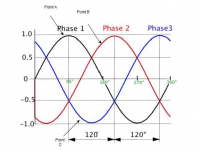Assume points A, B,C of some magnitude let us say MagA, MagB, MagC.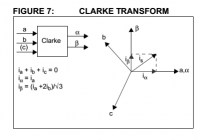where exactly they lie on the a,b,c axis. I mean to say i want to represent on the a,b,c axis.
Q3. And from the above diagram do the phase currents only have magnitude or do they have angle as well. For example for point A what is its angle? Please help.

Attachments

• microchip_01078B.pdf
474.9 KB · Views: 9

VSef

Newbie level 6I am referring to the application note attached. I am bit confused with the Clarke Transform the statement from the document page no 8 is
"The first coordinate transform, called the Clarke Transform, moves a three-axis, two-dimensional coordinate system, referenced to the stator, onto a
two-axis system, keeping the same reference (see Figure 7, where ia, ib and ic are the individual phase currents)."

Q1. How it is concluded it is two-dimensional coordinate system? I was assuming since it is having 3 currents it should be 3 dimensional.
Q2. I have created one 3 phase systemAssume points A, B,C of some magnitude let us say MagA, MagB, MagC.where exactly they lie on the a,b,c axis. I mean to say i want to represent on the a,b,c axis.
Q3. And from the above diagram do the phase currents only have magnitude or do they have angle as well. For example for point A what is its angle? Please help.

A1. It is a 2 axis system since the vectors are now presented by the alpha and beta axis (before the transformation they were presented by a, b and c)

A2. The vectors pulsate along the axis a-axis, b-axis and c-axis respectively depending on time, magnitude, frequency and phase. Check out this animation

A3. They do have angles which depends on the load impedence.

•electronicsman

electronicsman

Points: 2

electronicsman

Full Member level 5Thank you for the reply. I thought the angle is the x - axis on the graph like 0, 90 etc but confused when you say it is depending on load impedance. Can you please send me any link explaining that? I know i am missing lot of basics to understand all these things, I am trying to put all the things together and understand them. Meanwhile i also do not understand the following terms
"If this feature is enabled in the code, the software compensates for the voltage ripple on the DC bus. This in turn makes the hardware design economical by reducing the size of the buffer capacitor on the DC bus."
What exactly is this DC bus? Is it not the same as Vdc?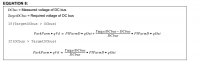- - - Updated - - -

I got the following information regarding the DC Bus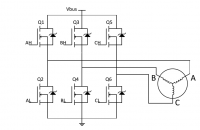"Vbus is a higher-voltage DC supply to power the motor." But what is ripple? How it happens and when it happens?

VSef

Newbie level 6When voltage is implied current is always dependent on the load impedance. I think you have to start by understanding about vector spaces and their usage in realizing inverters. See the following links:

https://theses.lib.vt.edu/theses/available/etd-2798-1216/unrestricted/chap2.pdf

As for your 2nd question, the ripple observed on the DC bus will degrade the output quality of your inverter. You can either increase your input cap size or try to compensate for the ripples with digital techniques. I prefer the 1st option but do know there are techniques to help you to compensate for the ripple

•electronicsman

electronicsman

Points: 2

electronicsman

Full Member level 5Yes I am not clear in my requirements. As per the document I want little clarity on the following statement it will help me to understand little better
"Considering an electric drive system as shown in Figure 1, the controller generates a reference voltage, us, represented with voltage space vector, as equation ( 1 )."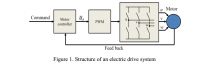The statement the controller generates reference voltage regarding this
Q1, On what basis does it generate the reference voltage?
My assumption to this question is that i need to generate a sine wave ( 3 sine waves ?) which are 120 degrees apart for PMSM motor. The amplitude and frequency of the sine wave defines the speed of the motor. And need to control the speed and frequency of the reference voltage based on the rotor position and speed. Am I correct on this? Once I get the reference voltage I determine the duty cycles using the SVPWM and apply to the legs? Please help.

Status
Not open for further replies.